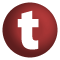# Ordering

#### trait Ordering[T] extends Comparator[T] with PartialOrdering[T]

A trait for representing total orderings. It is important to distinguish between a type that has a total order and a representation of total ordering on some type. This trait is for representing the latter.

A total ordering is a binary relation on a type `T` that is also an equivalence relation and partial ordering on values of type `T`. This relation is exposed as the `compare` method of the `Ordering` trait. This relation must be:

• reflexive: `compare(x, x) == 0`, for any `x` of type `T`.
• symmetry: `compare(x, y) == z` and `compare(y, x) == w` then `math.signum(z) == -math.signum(w)`, for any `x` and `y` of type `T` and `z` and `w` of type `Int`.
• transitive: if `compare(x, y) == z` and `compare(y, w) == v` and `math.signum(z) >= 0` and `math.signum(v) >= 0` then `compare(x, w) == u` and `math.signum(z + v) == math.signum(u)`, for any `x`, `y`, and `w` of type `T` and `z`, `v`, and `u` of type `Int`.

Go to: companion

### Inherits

1. PartialOrdering
2. Equiv
3. Comparator
4. AnyRef
5. Any

### Value Members

1. #### def compare(x: T, y: T): Int

Returns a negative integer iff `x` comes before `y` in the ordering, returns 0 iff `x` is the same in the ordering as `y`, and returns a positive number iff `x` comes after `y` in the ordering

Returns a negative integer iff `x` comes before `y` in the ordering, returns 0 iff `x` is the same in the ordering as `y`, and returns a positive number iff `x` comes after `y` in the ordering.

attributes: abstract
Go to: companion
2. #### def equals(arg0: Any): Boolean

This method is used to compare the receiver object (`this`) with the argument object (`arg0`) for equivalence

This method is used to compare the receiver object (`this`) with the argument object (`arg0`) for equivalence.

The default implementations of this method is an equivalence relation:

• It is reflexive: for any instance `x` of type `Any`, `x.equals(x)` should return `true`.
• It is symmetric: for any instances `x` and `y` of type `Any`, `x.equals(y)` should return `true` if and only if `y.equals(x)` returns `true`.
• It is transitive: for any instances `x`, `y`, and `z` of type `AnyRef` if `x.equals(y)` returns `true` and `y.equals(z)` returns `true`, then `x.equals(z)` should return `true`.

If you override this method, you should verify that your implementation remains an equivalence relation. Additionally, when overriding this method it is often necessary to override `hashCode` to ensure that objects that are "equal" (`o1.equals(o2)` returns `true`) hash to the same `Int` (`o1.hashCode.equals(o2.hashCode)`).

arg0

the object to compare against this object for equality.

returns

`true` if the receiver object is equivalent to the argument; `false` otherwise.

definition classes: AnyRef ⇐ Any
Go to: companion
3. #### def equiv(x: T, y: T): Boolean

Returns `true` iff `x` is equivalent to `y` in the ordering

Returns `true` iff `x` is equivalent to `y` in the ordering.

Go to: companion
4. #### def gt(x: T, y: T): Boolean

Returns `true` iff `y` comes before `x` in the ordering and is not the same as `x`

Returns `true` iff `y` comes before `x` in the ordering and is not the same as `x`.

Go to: companion
5. #### def gteq(x: T, y: T): Boolean

Returns `true` iff `y` comes before `x` in the ordering

Returns `true` iff `y` comes before `x` in the ordering.

Go to: companion
6. #### def hashCode(): Int

Returns a hash code value for the object

Returns a hash code value for the object.

The default hashing algorithm is platform dependent.

Note that it is allowed for two objects to have identical hash codes (`o1.hashCode.equals(o2.hashCode)`) yet not be equal (`o1.equals(o2)` returns `false`). A degenerate implementation could always return `0`. However, it is required that if two objects are equal (`o1.equals(o2)` returns `true`) that they have identical hash codes (`o1.hashCode.equals(o2.hashCode)`). Therefore, when overriding this method, be sure to verify that the behavior is consistent with the `equals` method.

definition classes: AnyRef ⇐ Any
Go to: companion
7. #### def lt(x: T, y: T): Boolean

Returns `true` iff `x` comes before `y` in the ordering and is not the same as `y`

Returns `true` iff `x` comes before `y` in the ordering and is not the same as `y`.

Go to: companion
8. #### def lteq(x: T, y: T): Boolean

Returns `true` iff `x` comes before `y` in the ordering

Returns `true` iff `x` comes before `y` in the ordering.

Go to: companion
9. #### def max(x: T, y: T): T

Returns the argument which comes later in the ordering

Returns the argument which comes later in the ordering.

Go to: companion
10. #### def min(x: T, y: T): T

Returns the argument which comes earlier in the ordering

Returns the argument which comes earlier in the ordering.

Go to: companion

12. #### def on[U](f: (U) ⇒ T): Ordering[U]

Given a function U => T, creates Ordering[U]

Given a function U => T, creates Ordering[U].

Go to: companion

14. #### def toString(): String

Returns a string representation of the object

Returns a string representation of the object.

The default representation is platform dependent.

definition classes: AnyRef ⇐ Any
Go to: companion
15. #### def tryCompare(x: T, y: T): Some[Int]

An Ordering is defined at all x and y

An Ordering is defined at all x and y.

Go to: companion